français | español | polski | 日本語 |

Pokemon Math 168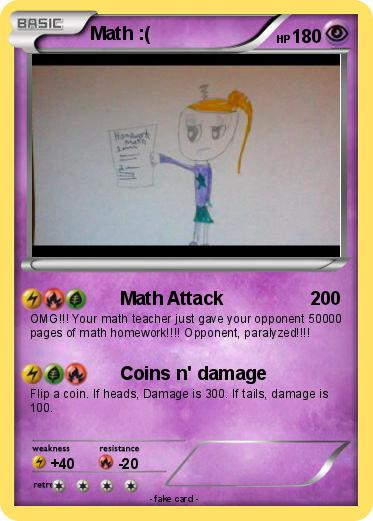Language Card : english

Creation Date : 25 February 2017

### Pokemon Passport

Name : Math :(

Serie : Black & White

Type : Psychic

Attack 1 : Math Attack
OMG!!! Your math teacher just gave your opponent 50000 pages of math homework!!!! Opponent, paralyzed!!!!

Attack 2 : Coins n' damage
Flip a coin. If heads, Damage is 300. If tails, damage is 100.

### Vote for this card

(1 vote)

Related cards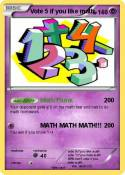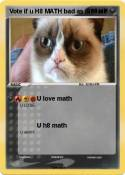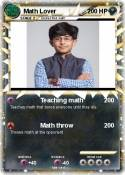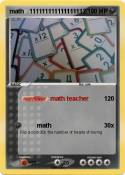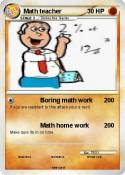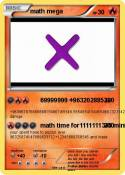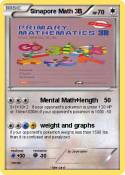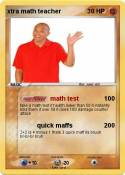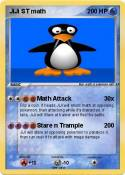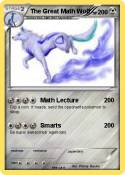Foxeh the math Fox | Khan academy | Vote 5 if you hate math | Math Mann | Ratey the Math Cat | I Can Do Math Everyday! | MATH | Math Teacher | MATh | math team | Math Book | Math knight | Brice | Math | Math Teacher | math 100 x | me in math | Math man | Daniel EX | Albert Einstein | MATH | Nerd | math | Math Homework | Cool Math | math doesn't lie | Tushar | Math 24 | Math Knight | Math Man | Math | Boring Math Teacher | Math | Mrs Ferriola | 1+1 | Ratey hte math cat | St Math | Mrs Baker | baldi | Math | Math | Math | Math | Math | more math | math | Asian guy | Asian guy | School | Math | prodigy guy | xtramath guy | Math Quiz | Vote if you like math | Matt's math degree | ??????????????? | njo | Math | Pi | ??? | math | PIE | can you Math????? | math | Mr. C | Math | math pig | connor | math | M I C H A L